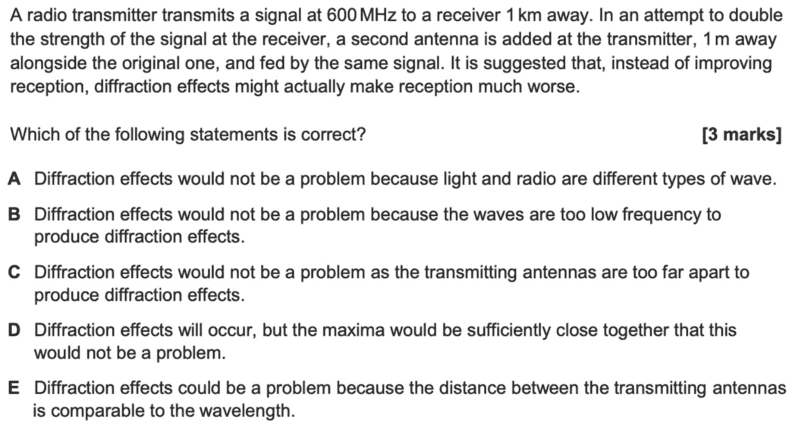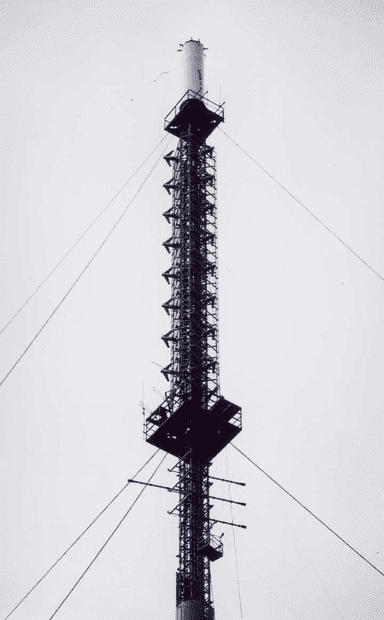# Do Radio Transmitters cause diffraction?

phantomvommand
Homework Statement:
Relevant Equations:
No equations, but qualitative understanding needed.I am very confused as to how diffraction even happens. I am thinking that radio transmitters are those tall towers. (My country doesn't have those, our lines are underground).

Where is the 2nd antenna, and how does the diagram look like?

Does it look like: (The dashes are to fill up space)
-------------Antenna 1
-------------Antenna 2

The question seems to suggest that the distance between the 2 antennas is the 'slit width', through which radio waves (from some other source) pass through and get diffracted.

However, I am thinking that the antenna is the source of radio waves, meaning that they are the 'slits' in a 2-slit interference setup, and thus any diffraction should be due to the 'slit width', which is inherent to each antenna, and not dependent on the 2nd antenna.

Am I wrong?

Also, because the question mentions some 'problem with signal strength due to diffraction', am I right to say that the problem is that of reduced intensity detected?

The answer to the question is E.

•Delta2

Homework Helper
Gold Member
2022 Award
Does it mean interference, rather than diffraction?

Did you calculate the wavelength?

•phantomvommand and hutchphd
phantomvommand
Does it mean interference, rather than diffraction?

Did you calculate the wavelength?
The wavelength is 0.5m.
if it meant interference, the receiver would get a signal at maximum amplitude, by virtue of the fact that it is at the central maxima.

I should note that the qn says “the 2nd antenna is placed alongside”, which could mean that the intended setup is one where the 2 antennas and the receiver are all along the same line. But even in this case, the path difference of 1m is = 2 lambda, so the signal detected is still maximum, and there is no problem caused by interference.

Since there is still some problem, I suppose the question must mean diffraction as per what it states; interference causes no problems.

Homework Helper
Gold Member
2022 Award
I should note that the qn says “the 2nd antenna is placed alongside”, which could mean that the intended setup is one where the 2 antennas and the receiver are all along the same line. But even in this case, the path difference of 1m is = 2 lambda, so the signal detected is still maximum, and there is no problem caused by interference.

Since there is still some problem, I suppose the question must mean diffraction as per what it states; interference causes no problems.

Wouldn't interference depend on how the two antennas and the receiver are arranged?

•phantomvommand
Homework Helper
Gold Member
2022 Award
PS I think it is using diffraction to mean the effects of interference.

•phantomvommand
phantomvommand
PS I think it is using diffraction to mean the effects of interference.
Then how is the answer E? E strongly suggests diffraction means diffraction; interference effects can happen regardless of whether the distance between the antenna is comparable to the wavelength.

Homework Helper
Gold Member
2022 Award
Then how is the answer E? E strongly suggests diffraction means diffraction; interference effects can happen regardless of whether the distance between the antenna is comparable to the wavelength.
Not really. If two sources are ##1m## apart, then the difference in path length to any point is at most ##2m##. If ##2m## is small compared to the wavelength, then interference effects will be negligible everywhere.

•phantomvommand
phantomvommand
Not really. If two sources are ##1m## apart, then the difference in path length to any point is at most ##2m##. If ##2m## is small compared to the wavelength, then interference effects will be negligible everywhere.
Isn't the difference in path length at most 1m?

Antenna 1 ---1m--- Antenna 2 ----------- Receiver

2m (or 1m) is not small compared to the wavelength = 0.5m. I suppose you are supposed to interpret "alongside" as anywhere, instead of on the line between Antenna 1 and Receiver?

Homework Helper
Gold Member
2022 Award
Isn't the difference in path length at most 1m?

Antenna 1 ---1m--- Antenna 2 ----------- Receiver

2m (or 1m) is not small compared to the wavelength = 0.5m. I suppose you are supposed to interpret "alongside" as anywhere, instead of on the line between Antenna 1 and Receiver?
Yes, ##1m##. I was thinking ##\pm 1m##.

Yes, E says "could be a problem" which suggests you'd need to know precisely where the receiver is to avoid destructive interference. Or destructive diffraction, if you prefer!

•phantomvommand
rsk
It's hard to be sure (and for me, odd to see 3 marks for a multiple choice question) but it seems this is asking you to think about the double source / double slit equation with the two antennae being the sources and the "fed by the same signal" being the "coherent sources" bit.

BUT using that logic, I don't see an answer I particularly like. E is closest, because the fringe width or separation would be large enough that you might easily have your receiver in the wrong place.

I agree though it seems to be using diffraction where it means interference. Students often confuse these terms but it's not surprising given how frequently the term "diffraction pattern" is used.

•phantomvommand
Gold Member
In classical physics diffraction and interference are really the same thing. So I wouldn't get too hung up on those words. It maybe a bit beyond this course level, but you could look into Huygens' Principle to see how the calculations are really the same. However they are generated, if you have multiple wave sources at the same frequency, you have to consider interference when you solve for the field intensity at distant points.

•rsk and phantomvommand
Gold Member
Homework Statement:: Please see attached picture.
Relevant Equations:: No equations, but qualitative understanding needed.

I am very confused as to how diffraction even happens. I am thinking that radio transmitters are those tall towers. (My country doesn't have those, our lines are underground).
You are not the only one who's confused. What is the context of your question? If "those tall towers" are used for UHF TV transmission then the transmitting antenna will be at the top and that sort of antenna will have a number of elements. (This array has many dipoles, spaced vertically and horizontally)If you hadn't been told about the types of antennae have been used then you would have to assume that they are simple vertical dipoles. In the horizontal plane, they would each have an omnidirectional pattern (they are point sources). At a large distance (the calculations are easiest at 'infinity') the signals from each will add in a way that depends on the amplitude and relative phase. Amplitudes can be assumed equal all round a large radius circle and the resultant will just depend on the relative phases - which depends on the path difference from each source. This is just like the Two slits but these slits are very near each other, compared to Young's slits. Also, the pattern will cover 360 degrees and not just a projection screen.
Any system of waves will always have a 'diffraction' pattern but this is so simple that the diffraction pattern boils down to a simple interference pattern because the two sources each have 'zero size' and you can just sum the contributions instead of integrating over finite source widths.
As for maxes and mins, any direction in which the path lengths are equal will produce 2A resultant and any direction where the two path lengths are different by a half wavelength will have cancellation. In the broadside direction they will always add to give 2A, whatever the spacing. In your case, there will also be a max in the line of the 4λ spaced elements. Do some trigonometry and find other possible directions for maxima. In between the directions of the maxima, there will be null directions.
4λ would not usually be chosen for a practical system - just for giving students a hard time!

interference causes no problems.
Hmm. The whole thing works by interference - that's not a problem.

•rsk and phantomvommand